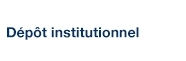# Temporal Accumulation of First-Order Linearization Error for Semi-Lagrangian Passive Advection

Tanguay, Monique; Polavarapu, Saroja et Gauthier, Pierre (1997). « Temporal Accumulation of First-Order Linearization Error for Semi-Lagrangian Passive Advection ». Monthly Weather Review, 125(6), pp. 1296-1311.

Fichier(s) associé(s) à ce document :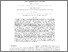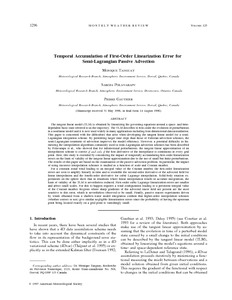Prévisualisation
PDF
Télécharger (345kB)

## Résumé

The tangent linear model (TLM) is obtained by linearizing the governing equations around a space- and time-dependent basic state referred to as the trajectory. The TLM describes to first-order the evolution of perturbations in a nonlinear model and it is now used widely in many applications including four-dimensional data assimilation. This paper is concerned with the difficulties that arise when developing the tangent linear model for a semi-Lagrangian integration scheme. By permitting larger time steps than those of Eulerian advection schemes, the semi-Lagrangian treatment of advection improves the model efficiency. However, a potential difficulty in linearizing the interpolation algorithms commonly used in semi-Lagrangian advection schemes has been described by Polavarapu et al, who showed that for infinitesimal perturbations, the tangent linear approximation of an interpolation scheme is correct if and only if the first derivative of the interpolator is continuous at every grid point. Here, this study is extended by considering the impact of temporally accumulating first-order linearization errors on the limit of validity of the tangent linear approximation due to the use of small but finite perturbations. The results of this paper are based on the examination of the passive advection problem. In particular, the impact of using incorrect interpolation schemes is studied as a function of scale and Courant number. For a constant zonal wind leading to an integral value of the Courant number, the first-order linearization errors are seen to amplify linearly in time and to resemble the second-order derivative of the advected field for linear interpolation and the fourth-order derivative for cubic Lagrange interpolation. Solid-body rotation experiments on the sphere show that in situations where linear interpolation results in accurate integrations, the limit of validity of the TLM is nevertheless reduced. First-order cubic Lagrange linearization errors are smaller and affect small scales. For this to happen requires a wind configuration leading to a persistent integral value of the Courant number. Regions where sharp gradients of the advected tracer field are present are the most sensitive to this error, which is nevertheless observed to be small. Finally, passive tracers experiments driven by winds obtained from a shallow-water model integration confirm that higher-order interpolation schemes (whether correct or not) give similar negligible linearization errors since the probability of having the upstream point being located exactly on a grid point is vanishingly small.

Type: Article de revue scientifique © Copyright  AMS https://www.ametsoc.org/ams/index.cfm/publications/authors/journal-and-bams-authors/author-resources/copyright-information/copyright-policy/) Trajectory, Linearization, Numerical method, Advection, Lagrangian model, Interpolation, Error, Algorithm, Theoretical study, Tangent linear model Faculté des sciences > Département des sciences de la Terre et de l'atmosphère Pierre Gauthier 26 avr. 2016 14:25 26 mai 2016 14:18 http://archipel.uqam.ca/id/eprint/8296

## Statistiques

Voir les statistiques sur cinq ans...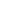RECHERCHER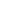PARCOURIR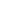LIBRE ACCÈS